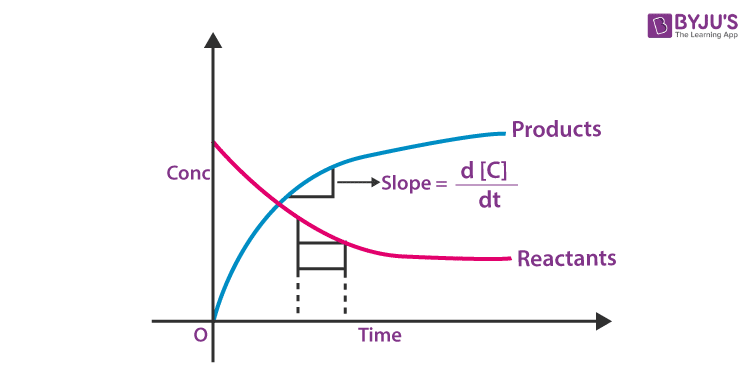Checkout JEE MAINS 2022 Question Paper Analysis : Checkout JEE MAINS 2022 Question Paper Analysis :

# Chemistry Practical Class 12 Effect of Concentration and Temperature on the Rate of Reaction between Sodium thiosulphate and Hydrochloric acid Viva Questions with Answers

Q1. Name the two solutions used in the experiment.

Answer: Sodium thiosulphate and hydrochloric acid.

Q2. What is the molecular formula of sodium thiosulphate?

Q3. What is the concentration of sodium thiosulphate used in this experiment?

Q4. What is the concentration of hydrochloric acid used in this experiment?

Q5. Write the equation for the reaction between sodium thiosulphate and hydrochloric acid.

Answer: Na2S2O3 + 2HCl → 2NaCl + SO2 + H2O + S

Q6. What will happen when dilute hydrochloric acid is added to sodium thiosulphate solution.

Answer: Yellow precipitate will be formed.

Q7. What is the yellow substance produced in sodium thiosulphate and hydrochloric acid reaction?

Q8. What is the effect of concentration on the rate of a reaction?

Answer: The rate of reaction increases with concentration. A higher concentration of a reactant leads to more collisions of reactant in a specific time, thereby increasing the reaction rate.

Q9. When should we start the stopwatch?

Answer: We should start the stopwatch after pouring half of the hydrochloric acid into the sodium thiosulphate solution flask.

Q10. What is the importance of concentrated nitric acid?

Answer: Concentrated nitric acid is used to wash the apparatus used in the experiment.

Q11. How will you plot a graph between the concentration and time of reaction?

Answer:Q12. What is the rate equation for a first-order reaction?

Answer: k = 2.303 / t log a / a-x

Q13. What are the factors that affect the rate of a reaction?

Answer: Various factors can affect the rate of a chemical reaction.

• Temperature
• Concentration of the reactant
• Physical state
• Catalyst

Q14. What is the law of mass action?

Answer: Law of Mass Action states that the rate of the chemical reaction is proportional to the product of the masses of the reacting substances, with each mass raised to a power equal to the coefficient that occurs in the chemical equation.

Q15. For which type of reactions, do order and molecularity have the same value?

Answer: Order and molecularity have the same value for elementary reactions taking place in a single step.

Q16. What is the effect of temperature on the rate of a reaction?

Answer: The rate of reaction increases with temperature. With the increasing temperature, the energy possessed by the reacting species increases. As a result, more species are in a position to cross the activation barrier. Therefore the reaction rate increases with a temperature rise.

Q17. Write the rate equation for the reaction 2A + B → C if the reaction order is zero.

Answer: The rate equation for the reaction 2A + B → C in the zero-order reaction is

Rate = k [A]0 [B]0 = k

Q18. Why can’t the molecularity of any reaction be equal to zero?

Answer: The molecularity of any reaction is the number of the reactant molecules or species colliding simultaneously in an elementary reaction. A minimum of one reactant is needed to initiate a chemical reaction. Therefore, the molecularity of the reaction cannot be zero.

Q19. Why is a reaction with a molecularity of more than three rare?

Answer: Reaction with a molecularity of more than three is rare because a simultaneous collision between more than three particles is rare.

Q20. What are the units of the rate of reaction?

Answer: Mol -1 L -1 sec -1

Download Class 12 Chemistry Viva questions on the Effect of Concentration and Temperature on the Rate of Reaction between Sodium thiosulphate and Hydrochloric acid by clicking on the button below.